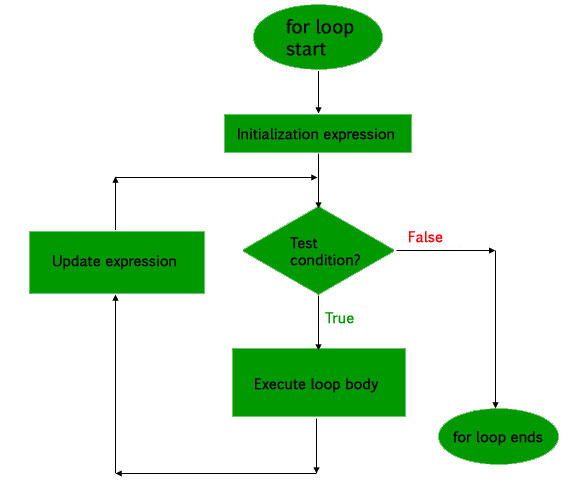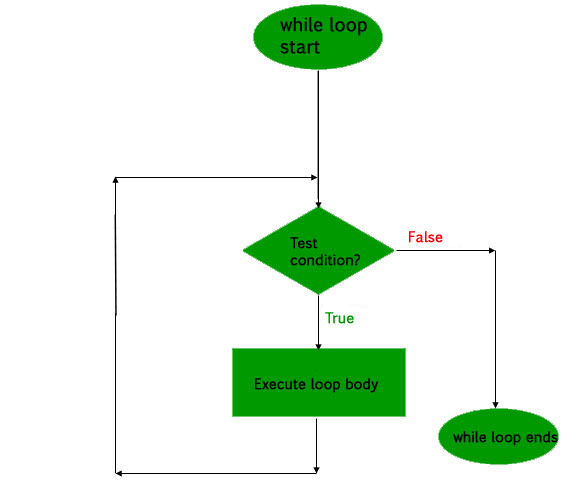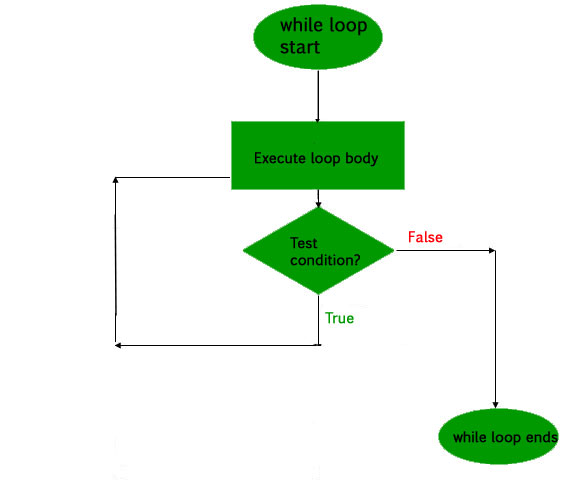Open In App
Related Articles
• Write an Interview Experience

# PHP | Loops

Like any other language, loop in PHP is used to execute a statement or a block of statements, multiple times until and unless a specific condition is met. This helps the user to save both time and effort of writing the same code multiple times.

PHP supports four types of looping techniques;

1. for loop
2. while loop
3. do-while loop
4. foreach loop

Let us now learn about each of the above mentioned loops in details:

1. for loop: This type of loops is used when the user knows in advance, how many times the block needs to execute. That is, the number of iterations is known beforehand. These type of loops are also known as entry-controlled loops. There are three main parameters to the code, namely the initialization, the test condition and the counter.

Syntax:

```for (initialization expression; test condition; update expression) {
// code to be executed
}
```

In for loop, a loop variable is used to control the loop. First initialize this loop variable to some value, then check whether this variable is less than or greater than counter value. If statement is true, then loop body is executed and loop variable gets updated . Steps are repeated till exit condition comes.

• Initialization Expression: In this expression we have to initialize the loop counter to some value. for example: \$num = 1;
• Test Expression: In this expression we have to test the condition. If the condition evaluates to true then we will execute the body of loop and go to update expression otherwise we will exit from the for loop. For example: \$num <= 10;
• Update Expression: After executing loop body this expression increments/decrements the loop variable by some value. for example: \$num += 2;

Example:

 ``

Output:

```1
3
5
7
9
```

Flow Diagram:2. while loop: The while loop is also an entry control loop like for loops i.e., it first checks the condition at the start of the loop and if its true then it enters the loop and executes the block of statements, and goes on executing it as long as the condition holds true.

Syntax:

```while (if the condition is true) {
// code is executed
}
```

Example:

 ``

Output:

```4
6
8
10
12
```

Flowchart:3. do-while loop: This is an exit control loop which means that it first enters the loop, executes the statements, and then checks the condition. Therefore, a statement is executed at least once on using the do…while loop. After executing once, the program is executed as long as the condition holds true.

Syntax:

```do {

//code is executed

} while (if condition is true);
```

Example:

 ``

Output:

```4
6
8
10
12
```

This code would show the difference between while and do…while loop.

 ``

Output:

```The code is executed at least once
```

Flowchart:4. foreach loop: This loop is used to iterate over arrays. For every counter of loop, an array element is assigned and the next counter is shifted to the next element.
Syntax:

```foreach (array_element as value) {
//code to be executed
}
```

Example:

 ``

Output:

```10
20
30
40
50
60
Ram
Laxman
Sita
```

This article is contributed by Chinmoy Lenka. If you like GeeksforGeeks and would like to contribute, you can also write an article using contribute.geeksforgeeks.org or mail your article to contribute@geeksforgeeks.org. See your article appearing on the GeeksforGeeks main page and help other Geeks.